Question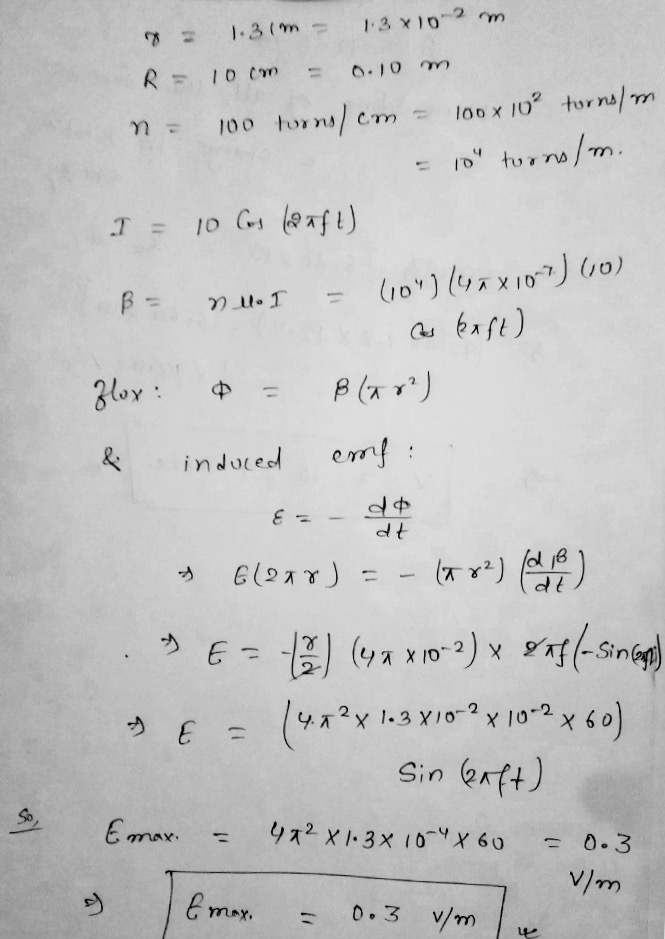#### Earn Coins

Coins can be redeemed for fabulous gifts.

Similar Homework Help Questions
• ### Problem 5. (4 points) Electric field induced by a changing magnetic field in a solenoid. long...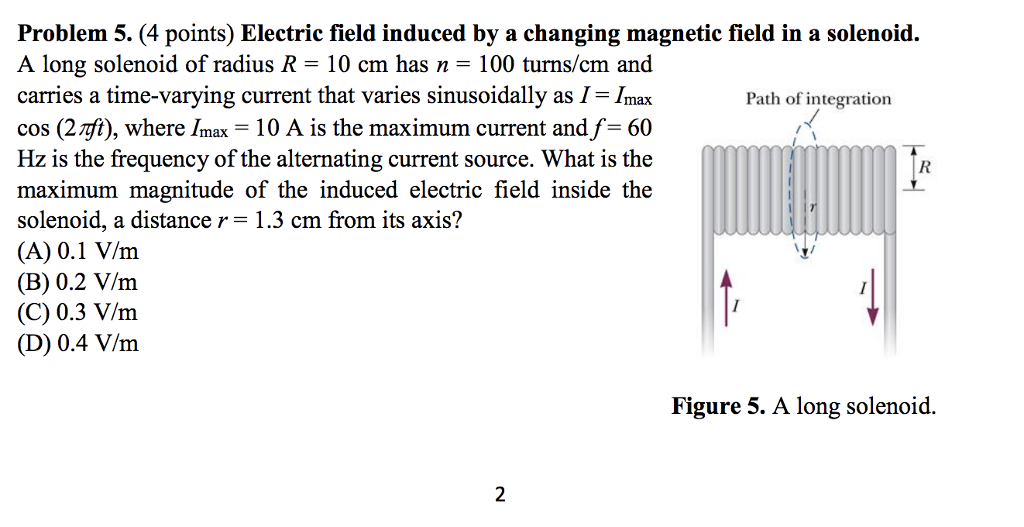Problem 5. (4 points) Electric field induced by a changing magnetic field in a solenoid. long solenoid of radius R = 10 cm has n = 100 turns/cm and carries a time-varying current that varies sinusoidally as I- Imax cos (2rft), where Imax- 10 A is the maximum current and f-60 Hz is the frequency of the alternating current source. What is the maximum magnitude of the induced electric field inside the solenoid, a distance r- 1.3 cm from its...

• ### 3. Find the magnitude of the induced electric field outside a long solenoid at a distance rZR from its central axis if the solenoid is of radius R and hasn turns of wire per unit length and carri...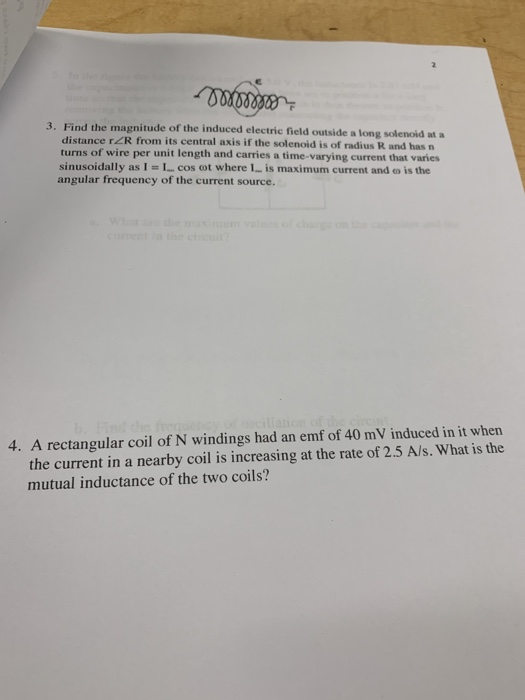3. Find the magnitude of the induced electric field outside a long solenoid at a distance rZR from its central axis if the solenoid is of radius R and hasn turns of wire per unit length and carries a time-varying current that varies sinusoidally as l = 1-cos cot where I-is maximum current and ω is the angular frequency of the current source. 4. A rectangular coil of N windings had an emf of 40 mV induced in it wher...

• ### 5) A solenoid comprises 500,000 turns of wire and is 10 cm long and 1.0 cm...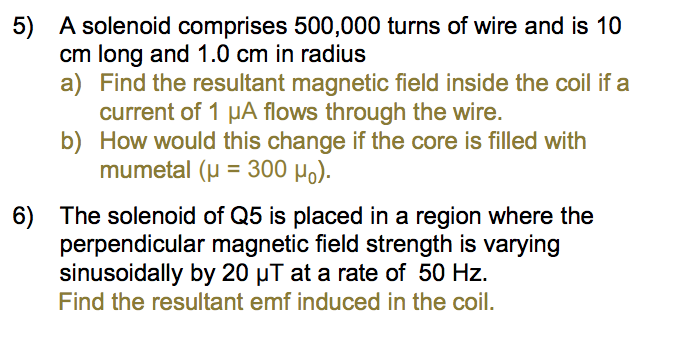5) A solenoid comprises 500,000 turns of wire and is 10 cm long and 1.0 cm in radius. a) Find the resultant magnetic field inside the coil if a current of 1 μA flows through the wire. b) How would this change if the core is filled with mumetal (μ = 300 μ0 ). 6) The solenoid of Q5 is placed in a region where the perpendicular magnetic field strength is varying sinusoidally by 20 μT at a rate of...

• ### Please solve all questions..thank you! solenoid of radius R-5 cm has n-10000 turns of wire per...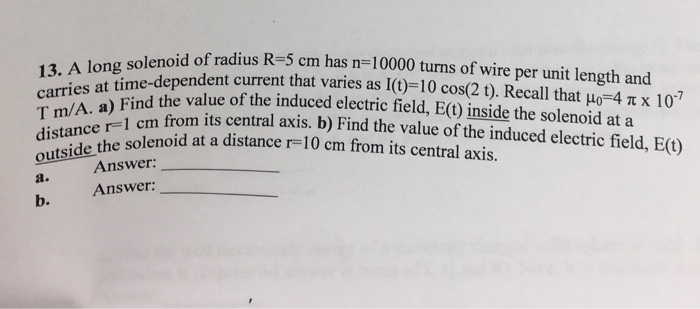Please solve all questions..thank you! solenoid of radius R-5 cm has n-10000 turns of wire per unit length and carries a Find the value of the induced electric field, E() inside the solenoid at a 13. A long e-dependent current that varies as l(t)-10 cos(2 t). Recall that μ-4 π x 107 T m/A. a) Find the w 1 cm from its central axis. b) Find the value of the induced electric field, E(U) r-1 c distane the solenoid at...

• ### A long solenoid with 1500 turns per meter and radius 1.6 cm carries an oscillating current...

A long solenoid with 1500 turns per meter and radius 1.6 cm carries an oscillating current I = 6.0 sin 100πt, where I is in amperes and t is in seconds. What is the maximum value of the electric field induced at a radius r = 1.2 cm from the axis of the solenoid.

• ### A small loop of area A = 4.2 mm2 is inside a long solenoid that has...

A small loop of area A = 4.2 mm2 is inside a long solenoid that has n = 413 turns/cm and carries a sinusoidally varying current i of amplitude 2.70 A and angular frequency 349 rad/s. The central axis of the loop and solenoid coincide. What is the amplitude of the emf induced in the loop?

• ### A solenoid of length 40 cm has 380 turns of radius 2.2 cm. A tightly wound...

A solenoid of length 40 cm has 380 turns of radius 2.2 cm. A tightly wound coil with 12 turns of radius 5.8 cm is placed around the solenoid. The axes of the coil and solenoid coincide. Find the emf induced in the coil if the current in the solenoid varies according to I=5.8sin(50πt) A. Answer: ______ cos(50πt) mV

• ### 1. A solenoid has a radius of R. It is long enough that it is reasonable to approximate the B-fie...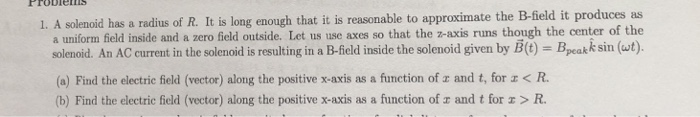1. A solenoid has a radius of R. It is long enough that it is reasonable to approximate the B-field it produces as a uniform field inside and a zero field outside. Let us use axes so that the z-axis runs though the center of the solenoid. An AC current in the solenoid is resulting in a B field inside the solenoid given by E (t Bp kk sin (wt (a) Find the electric field (vector) along the positive x-axis...

• ### An ideal solenoid carries a constant current. The solenoid has 50 turns/cm. There is an electron...

An ideal solenoid carries a constant current. The solenoid has 50 turns/cm. There is an electron moving in a perpendicular plane to the solenoid along a circular path with a radius of 1.5cm. The electron is moving at 0.025c. c = 2.998x10^8 m/s a) what is the angular frequency of the electrons motion b) what is the current in the solenoid c) what is the magnitude of the magnetic field in the solenoid

• ### Long solenoid has 210 turns/cm and carries a current i = 1.5A; its radius is 1.8...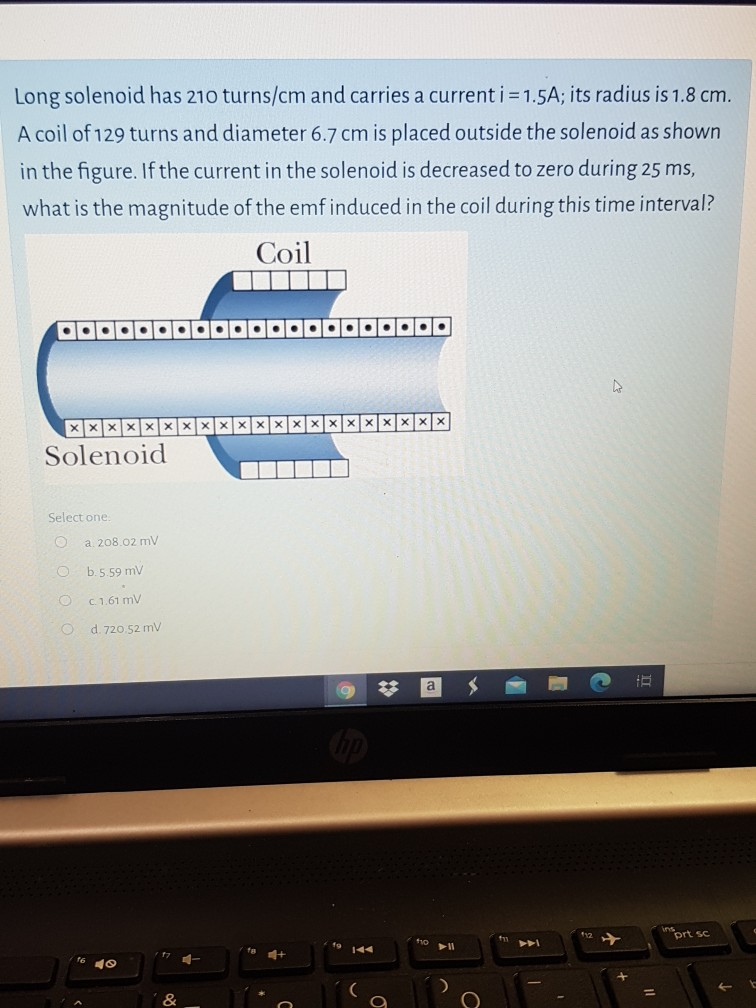Long solenoid has 210 turns/cm and carries a current i = 1.5A; its radius is 1.8 cm. A coil of 129 turns and diameter 6.7 cm is placed outside the solenoid as shown in the figure. If the current in the solenoid is decreased to zero during 25 ms, what is the magnitude of the emf induced in the coil during this time interval? Coil OO Solenoid Select one a 208.02 mv C 5.5.59 mv OC 1.61 mv O d....